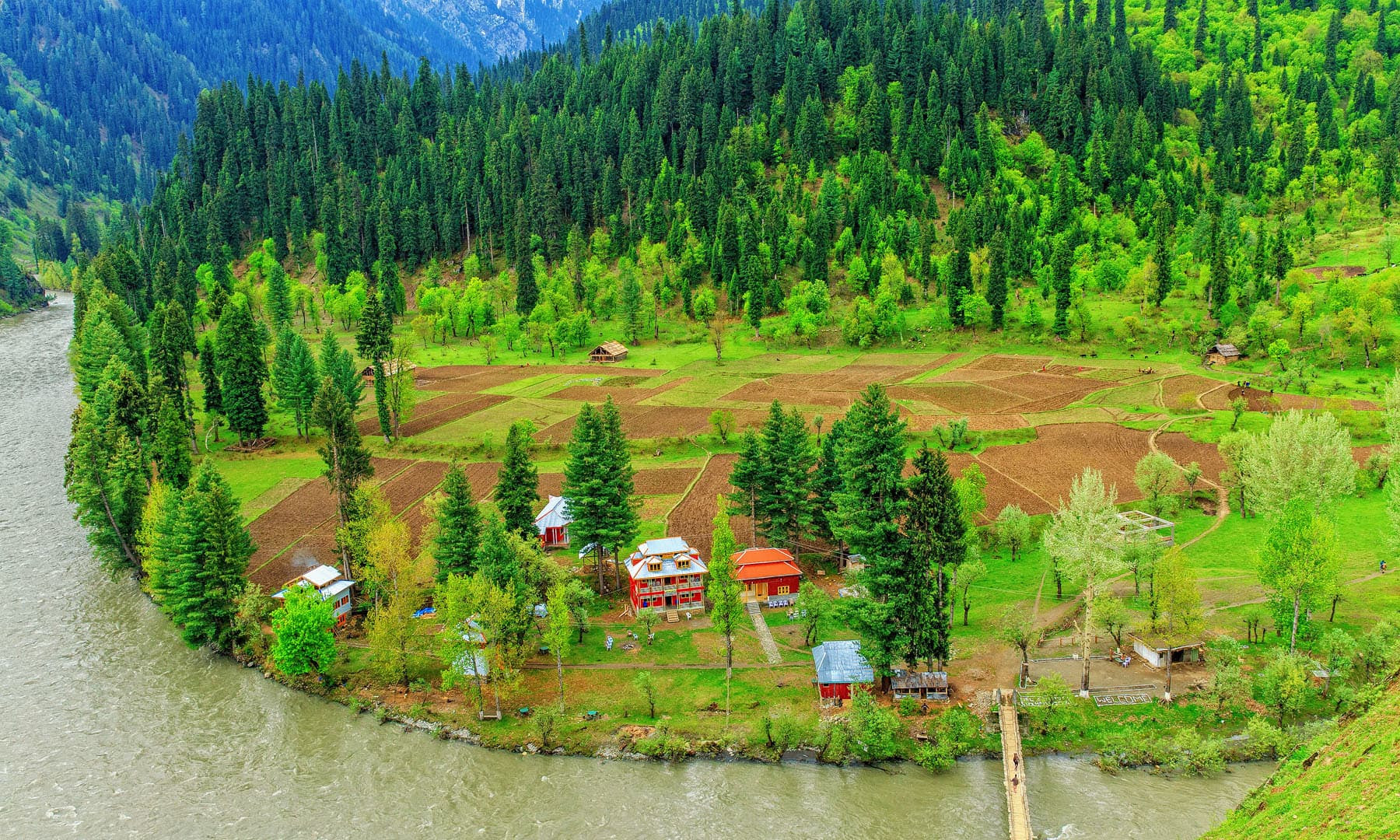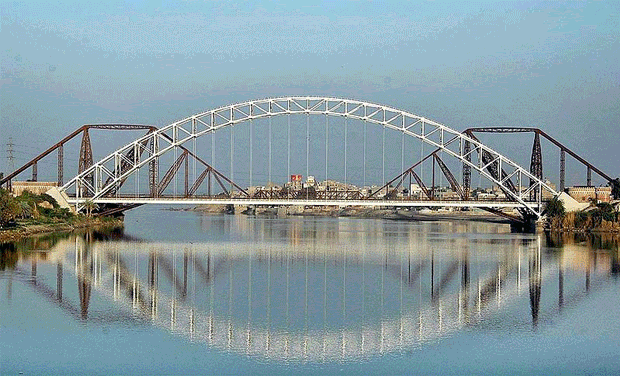Pakistans Rivers Mcqs | With

Answer Key | 2018 |

1) How many rivers flow in Punjab Province?

A. Six
B. Seven
C. Eight
D. Five
Answer is = D

2) How many rivers flow in Sindh Province?

A. Four
B. Seven
C. Eight
D. Nine
Answer is = A

3) How many rivers flow in Balochistan Province?

A. Six
B. Seven
C. Eight
D. Nine
Answer is = B

4) How many rivers flow in Khyber Pakhtunkhwa Province?

A. Six
B. Seven
C. Eight
D. Nine
Answer is = C

5) How many rivers are present in Pakistan?

A. 20
B. 22
C. 23
D. 24
Answer is = D6) Where Jehlum and Chenab rivers meet?

A. Rasol Pur
B. Panjnad
C. Trimmu
D. Khanki Headworks
Answer is = C

7) Where all rivers of Punjab enter into Indus?

A. Rasol Pur
B. Panjnad
C. Kot mitthan
D. Khanki Headworks
Answer is = C

8) Indus River is also known as

A. Abaseen River
B. Attock River
C. Skardu River
D. All of above
Answer is = D

9) Name the logest river of Pakistan?

A. Indus
B. Kabul
C. Chenab
D. Sutlej
Answer is = A

10) Which is the smallest river of Pakistan?

A. Indus
B. Ravi
C. Chenab
D. Sutlej
Answer is = B11) Which is the longest river in the sub continent?

A. Indus
B. Kabul
C. Barhamputra
D. Sutlej
Answer is = C

12) Name the most polluted river of Pakistan?

A. Indus
B. Ravi
C. Chenab
D. Sutlej
Answer is = B

13) Harrapa city is situated on the bank of

A. Indus
B. Ravi
C. Chenab
D. Sutlej
Answer is = B

14) Name the river in Pakistan whose annual flow is twice that of the Nile?

A. Indus
B. Kabul
C. Chenab
D. Sutlej
Answer is = A

15) From where the Indus River rises ?

A. Indus
B. Kabul
C. Tibet
D. Sutlej
Answer is = C16) Where the Indus River ends?

A. Indus
B. Kabul
C. Arabean sea
D. Sutlej
Answer is = C

17) Most of the country rivers flow into

A. Indus
B. Kabul
C. Chenab
D. Sutlej
Answer is = A

18) The whole agriculture of Pakistan depends on the water of which river?

A. Indus
B. Kabul
C. Chenab
D. All of above
Answer is = D

19) The Bari Doab cannal originates from the river

A. Indus
B. Kabul
C. Ravi
D. Sutlej
Answer is = C

20) Into which the Kabul River falls?

A. Indus
B. Kabul
C. Chenab
D. Sutlej
Answer is = A21) Name the tributaries of the Indus in the Punjab region

A. Indus
B. Kabul
C. Chenab
D. All of above
Answer is = D

22) Which of the following rivers is called “Nile of Pakistan” ?

A. Indus
B. Kabul
C. Chenab
D. Sutlej
Answer is = A

23) Panjkora River is located in the province of

A. Punjab
B. Sindh
C. Khyber Pakhtunkhwa
D. Balochistan
Answer is =C

24) Bolan River is located in the Province of

A. Punjab
B. Sindh
C. Khyber Pakhtunkhwa
D. Balochistan
Answer is = D

25) Baran River is located in the Province of

A. Punjab
B. Sindh
C. Khyber Pakhtunkhwa
D. Balochistan
Answer is = B26) Dast River is located in the Province of

A. Punjab
B. Sindh
C. Khyber Pakhtunkhwa
D. Balochistan
Answer is = D

27) Porali River is located in the Province of

A. Punjab
B. Sindh
C. Khyber Pakhtunkhwa
D. Balochistan
Answer is = D

28) Gomal and Kuram rivers are in the Province of

A. Punjab
B. Sindh
C. Khyber Pakhtunkhwa
D. Balochistan
Answer is = C

29) Rukshan river flows in the Province of

A. Punjab
B. Sindh
C. Khyber Pakhtunkhwa
D. Balochistan
Answer is = D

30) Kabul River is located in the Province

A. Punjab
B. Sindh
C. Khyber Pakhtunkhwa
D. Balochistan
Answer is = C31) What is the total length of Jehlum River?

A. 725 km
B. 254 km
C. 123 km
D. 785 km
Answer is = A

32) What is the total length of Ravi River?

A. 715 km
B. 254 km
C. 123 km
D. 785 km
Answer is = A

33) What is the total length of Indus River?
A. 2900 km
B. 2514 km
C. 1232 km
D. 7854 km
Answer is = A